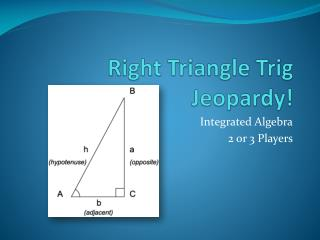DownloadDownload PresentationRight Triangle Trig Jeopardy!

# Right Triangle Trig Jeopardy!

Télécharger la présentation## Right Triangle Trig Jeopardy!

- - - - - - - - - - - - - - - - - - - - - - - - - - - E N D - - - - - - - - - - - - - - - - - - - - - - - - - - -
##### Presentation Transcript

1. Right Triangle Trig Jeopardy! Integrated Algebra 2 or 3 Players

2. The first player chooses the question by clicking on it. The first player to answer correctly gets the points. Make sure to keep track of points earned. You will need to use your graphing calculator.

3. Trig Potpourri 300 If sin(B)=0.5, find the value of B in degrees. a. 60b. 30 c. 0.008d. 87

4. Trig Potpourri 300 Determine the height, x, of the building to the nearest foot. a. 106ft b. 116ft c. 536ft d. 117ft

5. Trig Potpourri 300 A person standing on level ground is 2,000 feet away from the foot of a 420-foot-tall building, as shown in the accompanying diagram. To the nearest degree, what is the value of x? a. 12b. 2 c. 0 d. 78

6. Trig Potpourri 300 What is cos(A) in fraction form? a. b. c. d.

7. Trig Potpourri 300 Tanya runs diagonally across a rectangular field that has a length of 40 yards and a width of 30 yards, as shown in the diagram below. How far does she run to the nearest yard? a. 70ydsb. 50yds c. 2500ydsd. 500yds

8. Identify the Function 100 Which trigonometric function could be used to find the length of side PQ? a. cosc. arccos b. arcsind. sin

9. Identify the Function 200 Which trigonometric function could be used to find the measure of angle x ? a. sinb. tan c. arccosd. arcsin

10. Identify the Function 300 In right triangle XZY, which trigonometric function could be used to find the measure of angle x? a. arcsinb. cos c. tand. arctan

11. Identify the Function 400 Which is the correct equation needed to find the value of x? a. b. c. d.

12. Identify the Function 500 In triangle MTC, with right angle T, MC=85cm, CT=84cm, and TM = 13 cm. Which ratio represents the sine of C? a. b. c.d.

13. Whose Side are You On? 100 Find the value of x to the nearest whole number. a. 11b. 24 c. 5 d. 1

14. Whose Side are You On? 200 Find the value of x. a. 5inb. 25in c. 6in d. 26in

15. Whose Side are You On? 300 Find the length of the kite string. a. 30ftb. 65ft c. 78ftd. 32ft

16. Whose Side are You On? 400 A 30 foot long ramp is leaning against a wall at a construction site. If the ramp forms an angle of 32° with the ground, how high above the ground, to the nearest tenth, is the top of the ramp? a. 56.6ftb. 15.8ft c. 25.4ftd. 15.9ft

17. Whose Side are You On? 500 a. 52ft b. 48ft c. 57ft d. 43ft

18. What’s Your Angle? 100 Find the value of x to the nearest degree. a. 33 b. 56 c. 40d. 14

19. What’s Your Angle? 200 Find the value of x in degrees. a. 39b. 51 c. 9 d. 52

20. What’s Your Angle? 300 In the accompanying diagram, the base of a 15-foot ladder rests on the ground 4 feet from a 6-foot fence. If the ladder touches the top of the fence and the side of a building, what angle, to the nearest degree, does the ladder make with the ground? a. 34 b. 56 c. 24 d. 42

21. What’s Your Angle? 400 Ron and Francine are building a ramp for performing skateboard stunts, as shown in the accompanying diagram. The ramp is 7 feet long and 3 feet high. What is the measure of the angle, x, that the ramp makes with the ground, to the nearest tenth of a degree? a. 0.04b. 23.2 c. 64.6d. 25.4

22. What’s Your Angle? 500 The accompanying diagram shows a flagpole that stands on level ground. Two cables, r and s, are attached to the pole at a point 16 feet above the ground. The combined length of the two cables is 50 feet. If cable r is attached to the ground 12 feet from the base of the pole, what is the measure of the angle, x, to the nearest degree, that cable s makes with the ground? a. 58b. 32 c. 37d. 53

23. Pythagorean Theorem 100 Find the length of the missing side, x. Round to the nearest tenth. a.aa. 19.70 b. 19.6 c. 19.69 d. 19.7

24. Pythagorean Theorem 200 Find the length of the missing side, x. Round to the nearest hundredth. a. 15.7 b. 15.66 c. 15.65 d. 16

25. Pythagorean Theorem 300 The NuFone Communications Company must run a telephone line between two poles at opposite ends of a lake, as shown in the accompanying diagram. The length and width of the lake are 75 feet and 30 feet, respectively. What is the distance between the two poles, to the nearest foot? a. 80ft b. 68ft c. 81ft d. 69ft

26. Pythagorean Theorem 400 A cable 20 feet long connects the top of a flagpole to a point on the ground that is 16 feet from the base of the pole. How tall is the flagpole to the nearest foot? a. 8 ft b. 12 ft c. 10ft d. 26ft

27. Pythagorean Theorem 500 Which set of numbers could be the lengths of the sides of a right triangle? a. {10,24,26} b. {3,4,6} c. {12,16,30} d. {4,7,8}